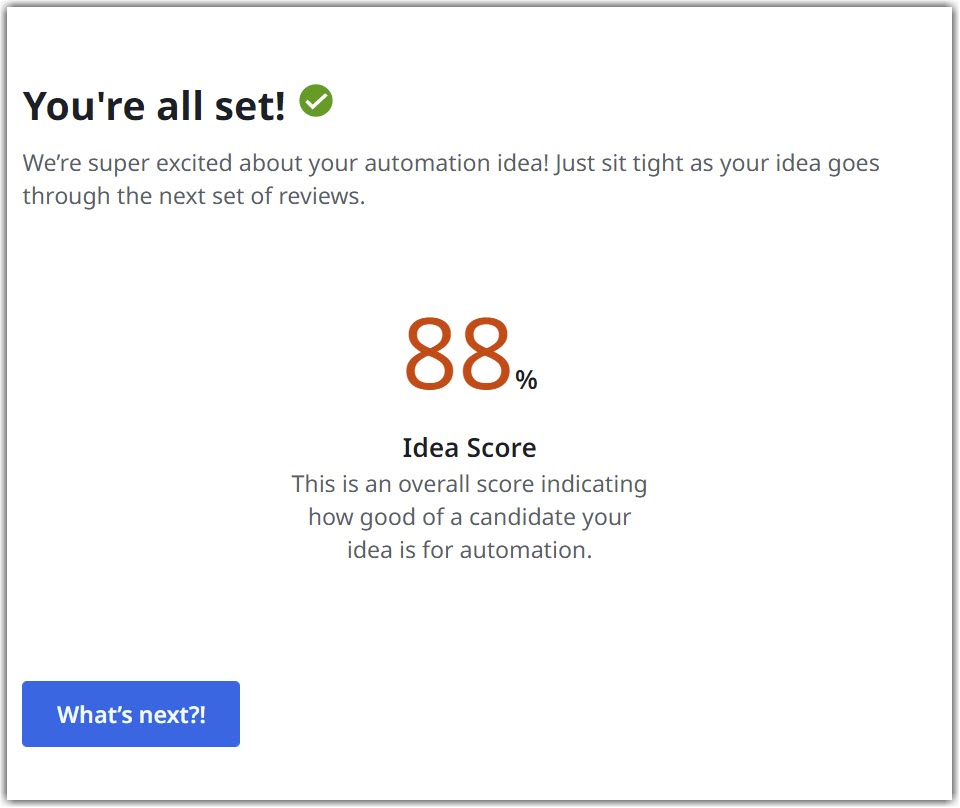Subscribe

# Information About the Idea Score

High- Level Scoring Algorithm

The Idea high-level scoring algorithm is implemented to vet out ideas that should not be automated.
Based on its value it's easy to separate Ideas based on their idea suitability and readiness and invest time in the ones that can bring value to your company.

Automation Hub uses the general factors applied to ideas algorithms. The difference is that it creates a guided experience available for anyone who wants to share their idea(s). Another differentiation is the fact that the exceptions are also implemented in the algorithm.

During the Idea submission process, an algorithm runs silently to compute the following output scores:

• Idea Score - expressed as % (from 0 to 100% - the higher the better).# Idea Score

This is the Idea overall score calculated as a simple arithmetic average between the following 5 factors ranked by the submitter on a Likert scale (from 1 to 5) or weighted average between suitability % and readiness % :

• The degree to which the process has rules;
• The Input's degree of digitization;
• Degree to which the input data is structured;
• The upcoming process changes;
• Existence of documentation describing the process/task.

### 📘Note:

All the above mentioned factors influence the result in a positive way the higher they are ranked.

# Suitability

This score helps with determining the extent to which an idea is fitted for automation and is calculated as a simple arithmetic average between the following 3 factors:

• Degree to which the process has rules;
• The Input's degree of digitization;
• Degree to which the input data is structured.

This score helps with determining the extent to which an idea is ready to be automated based on the process lifecycle. This is calculated as a simple arithmetic average between the following 2 factors:

• The upcoming process changes;
• Existence of documentation describing the process/task.Updated about a month ago